Contemporary Mathematics

# 12.2Graph Structures

Contemporary Mathematics12.2 Graph Structures

Figure 12.16 Neuroimaging shows brain activity. (credit: "MRI Scan" by NIH Image Gallery/Flickr, Public Domain)

### Learning Objectives

After completing this section, you should be able to:

1. Describe and interpret relationships in graphs.
2. Model relationships with graphs.

Graph theory is used in neuroscience to study how different parts of the brain connect. Neurobiologists use functional magnetic resonance imaging (fMRI) to measure levels of blood in different parts of the brain, called nodes. When nodes are active at the same time, it suggests there is a functional connection between them so they form a network. This network can be represented as a graph where the vertices are the nodes and the functional connections are the edges between them. (Mikey Taylor, "Graph Theory & Machine Learning in Neuroscience," Medium.com, June 24, 2020.

### Importance of the Degrees of Vertices

One reason scientists study these networks is to determine how successful the communication within a network continues to be when it experiences failures in nodes and connections. Graphs can be used to study the resilience of these networks. (Mikey Taylor, "Graph Theory & Machine Learning in Neuroscience," Medium.com, June 24, 2020)

### Example 12.6

#### Using Graphs to Understand Relationships

The graphs in Figure 12.17 represent neural networks, where the vertices are the nodes, and the edges represent functional connections between them. Which graph do you think would represent a network with the highest resistance to failure? Which graph would probably be the most vulnerable to failure? How might this relate to the degrees of the vertices?

Figure 12.17 Models of Neural Networks

Sociologists use graphs to study connections between people and to identify characteristics that make communities more resilient, more likely to stay connected over time. In the graphs shown, the edges represent social connections and the vertices are individuals.
Models of Communities
1.
Which graph do you think represents the most resilient community? What is the sum of the degrees of all the vertices in this graph?
2.
Which graph do you think represents the community that is least likely to stay connected over time? What is the sum of the degrees of all the vertices in this graph?
3.
Is the sum of the degrees higher or lower in a more resilient community?

### Relating the Number of Edges to the Degrees of Vertices

In the applications of graph theory to neuroscience and sociology in Example 12.6 and YOUR TURN 12.6, there was a correlation between the degrees of vertices and the resilience of a network. Researchers in many fields have also observed a direct relationship between the number of edges in a graph and the degrees of the vertices. To begin to understand this relationship, consider a graph with five vertices and zero edges as in Figure 12.18.

Figure 12.18 Graph with Five Vertices and zero Edges

Instead of being marked with a name, each vertex in Figure 12.18 is marked with its degree. In this case, all of the degrees are 0 so the sum of the degrees is also zero. Suppose that we add an edge between any two existing vertices and indicate the degrees of the vertices. This gives us a graph with five vertices and one edge like the graph in Figure 12.19.

Figure 12.19 Graph with Five Vertices and One Edge

Note that the degrees of two vertices increased, each by 1. So, the sum of the degrees is now 2. Suppose that we continue in this way, adding one edge at a time and making note of the number of edges and the sum of the degrees of the vertices as in Figure 12.20.

Figure 12.20 Comparing Number of Edges to Sum of Degrees

Figure 12.20 demonstrates a characteristic that is true of all graphs of any shape or size. When the number of edges is increased by one, the sum of the degrees increases by two. This happens because each edge has two ends and each end increases the degree of one vertex by one unit. As a result, the sum of the degrees of the vertices on any graph is always twice the number of edges. This relationship is known as the Sum of Degrees Theorem.

### FORMULA

For the Sum of Degrees Theorem,

$sum of the degrees=2×number of edgessum of the degrees=2×number of edges$

or

$number of edges=sum of degrees2number of edges=sum of degrees2$

### Example 12.7

#### Finding the Sum of Degrees

Suppose that a graph has five edges.

1. Find the sum of the degrees of the vertices.
2. Draw two different graphs that demonstrate this conclusion.

Suppose that the sum of the degrees of a graph is six.
1.
Find the number of edges.
2.
Draw two graphs that demonstrate your conclusion.

### Completeness

Suppose that there were five strangers in a room, A, B, C, D, and E, and each one would be introduced to each of the others. How many introductions are necessary? One way to begin to answer this question is to draw a graph with each vertex representing an individual in the room and each edge representing an introduction as in Figure 12.22.

Figure 12.22 Model of Introductions between Five Strangers

Let’s approach the problem by thinking about how many new people Person A would meet, then Person B, and so on, making sure not to repeat any introductions. The first graph in Figure 12.22 shows Person A meeting Persons B, C, D, and E, for a total of 4 introductions. The next graph shows that Person B still has to meet Persons C, D, and E, for a total of 3 more introductions. The next graph shows that Person C still has to meet Persons D and E, which is 2 more introductions. The next graph shows that Person D only remains to meet Person E, which is 1 more introduction. The final graph has $4+3+2+1=104+3+2+1=10$ edges representing 10 introductions. The last graph is an example of a complete graph because each pair of vertices is joined by an edge. Another way of saying this is that the graph is complete because each vertex is adjacent to every other vertex.Figure 12.23 shows complete graphs with three, four, five, and six vertices.

Figure 12.23 Complete Graphs with Up to Six Vertices

Suppose we want to know the number of introductions necessary in a room with six people. This would be represented by a complete graph with six vertices, and the total number of introductions would be $5+4+3+2+1=155+4+3+2+1=15$, the number of edges in the graph. In fact, you can always find the number of introductions in a room with $nn$ people by adding all the whole numbers from 1 to $n−1n−1$.

### FORMULA

The number of edges in a complete graph with $nn$ vertices is the sum of the whole numbers from 1 to $n−1n−1$, $1+2+3+⋯+(n−1)1+2+3+⋯+(n−1)$.

Suppose that we want to determine how many introductions are necessary in a room with 500 strangers. In other words, suppose that we want to determine the number of edges in a complete graph with 500 vertices. Adding up all the numbers from 1 to 499 could take a long time! In the next example, we use the Sum of Degrees Theorem to make the problem more manageable.

### Example 12.8

#### Using the Sum of Degrees Theorem

Use the Sum of Degrees Theorem to determine the number of introductions required in a room with

1. 6 strangers.
2. 10 strangers.
3. $nn$ strangers.

1.
Determine the number of introductions necessary in a room with 500 strangers using the Sum of Degrees Theorem.

Now we have a shorter way to calculate the number of introductions in a room with $nn$ strangers, and the number of edges on a complete graph with $nn$ vertices. Let’s update our formula.

### FORMULA

The number of edges in a complete graph with $nn$ vertices is $1+2+3+⋯+(n−1)=n(n−1)21+2+3+⋯+(n−1)=n(n−1)2$.

### Subgraphs

Sometimes a graph is a part of a larger graph. For example, the graph of South Florida Airports from Figure 12.7 is part of a larger graph that includes Orlando International Airport in Central Florida, which is shown in Figure 12.24

Figure 12.24 Orlando and South Florida Airports

The graph in Figure 12.24 includes an additional vertex, MCO, and additional edges shown with dashed lines. The graph of direct flights between South Florida airports from Figure 12.7 is called a subgraph of the graph that also includes direct flights between Orlando and the same South Florida airports in Figure 12.24. In general terms, if Graph B consists entirely of a set of edges and vertices from a larger Graph A, then B is called a subgraph of A.

### Example 12.9

#### Identifying a Subgraph

In Figure 12.25, Graph G is given, along with four diagrams. Determine whether each diagram is or is not a subgraph of Graph G and explain why.

Figure 12.25 Graph G and Potential Subgraphs

1.
Draw a subgraph of Graph F from Figure 12.3 that has exactly four vertices and five edges.

### Identifying and Naming Cycles

Figure 12.26 The water cycle begins and ends with water. (credit: "Diagram of the water cycle" by NASA, Public Domain)

When you think of a cycle in everyday life, you probably think of something that begins and ends the same way. For example, the water cycle (Figure 12.26) begins with water in a lake or ocean, which evaporates into water vapor, condenses into clouds, and then returns to earth as rain or some other form of precipitation that settles into lakes or oceans. A cycle in graph theory is similar in that it begins and ends in the same way: It is a series of connected edges that begin and end at the same vertex but otherwise never repeat any vertices.

In a cycle, there are always the same number of vertices as edges, and all vertices must be of degree 2. Cycles are often referred to by the number of vertices. For example, a cycle with 5 vertices can be called a 5-cycle. Cycles can also be named after polygons based on the number of edges. For example a 5-cycle is also called a pentagon. Table 12.1 lists these names for cycles of size 3 through size 10.

Cycle Category Number of Edges Example
triangle 3
pentagon 5
hexagon 6
heptagon 7
octagon 8
nonagon 9
decagon 10
Table 12.1 Categories of Cycles

There are many more polygon names, including a megagon that has a million edges and a googolgon that has $1010010100$ edges, but usually we just say $nn$-gon when the number $nn$ is past 10. For example, a cycle with 11 edges could be called an 11-gon.

Notice that the 10-cycle, or decagon, appears to cross over itself in Table 12.1. Remember, graphs can be drawn differently but represent the same connections. In Figure 12.27, the same decagon is transformed into a graph that does not appear to overlap itself. We have done this without changing any of the connections so both diagrams represent the same relationships, and both diagrams are considered decagons.

Figure 12.27 Transformation of a Decagon

### Who Knew?

Did the name "googolgon" ring a bell for you? If so, there is a good reason for it. The creators of Google.com renamed their search engine Google, a misspelled reference to the number "googol" alluding to the enormous number of calculations their algorithm can tackle. A googol is a 1 followed by 100 zeroes, or $1010010100$. (William L. Hosch, "Google, American Company," https://www.britannica.com/topic/Google-Inc, Encyclopedia Britannica online)

### Cyclic Subgraphs and Cliques

When cycles appear as subgraphs within a larger graph, they are called cyclic subgraphs. Cyclic subgraphs are named by listing their vertices sequentially. The vertex where you begin is not important. Graph K in Figure 12.28 has two triangle cycles (g, h, j) and (h, i, j), and one quadrilateral cycle (g, h, i, j).

Figure 12.28 Cycles in Graph K

### Checkpoint

The same cycle can be named in many ways, but we must keep in mind that listing two vertices consecutively indicates an edge exists between them. For example, (g, h, i, j) can also be named (h, i, j, g) or (j, i, h, g). However, you cannot name it (g, i, h, j,) which doesn't reflect the correct order of the vertices. We can see that the order (g, i, h, j) is incorrect because the cycle has no edges gi or hj.

### Example 12.10

#### Identifying Cyclic Subgraphs

Identify the types of cyclic subgraphs in Graph H in Figure 12.29 and name them.

Figure 12.29 Graph H

### Checkpoint

In order to avoid naming the same cycle twice, consider naming cycles beginning with the letter closest to the beginning of the alphabet and then progressing clockwise.

1.
Which types of cycles are in Graph G in Figure 12.32? Use the vertices of the graph to give a name for one of each type that you find.

We have seen that sociologists use graphs to study the structures of social networks. In sociology, there is a principle known as Triadic Closure. It says that if two individuals in a social network have a friend in common, then it is more likely those two individuals will become friends too. Sociologists refer to this as an open triad becoming a closed triad. This concept can be visualized as graphs in Figure 12.30. (Chakraborty, Dutta, Mondal, and Nath, "Application of Graph Theory in Social Media, International Journal of Computer Sciences and Engineering, 6(10):722-729) In the open triad in Figure 12.30, person a and person b each has a friendship with person c. In the closed triad, person a and person a have also developed a friendship. Notice that the graph representing the closed triad is a three-cycle, or a triangle, in graph theory terms.

Another topic of interest to sociologists, as well as computer scientists and scientists in many fields, is the concept of a clique. In a social group, a clique is a subgroup who are all friends. A triad is an example of a clique with three people, but there can be cliques of any size. In graph theory, a clique is a complete subgraph.

### Example 12.11

The graphs in YOUR TURN 12.6 represent social communities. The vertices are individuals and the edges are social connections. Use the graphs to answer the questions.

1. Which graph has the most triads? Name the triangles.
2. Which graph has the fewest triads? Name the triangles.
3. How might an increase in the number of triads in a graph affect the resilience of a community? Explain your reasoning.
4. Identify a clique with more than three vertices in Graph E by naming its vertices.

1.
From Exercise 35 in Section 12.1 Exercises, Chloe is interested to know how many in her network of Roblox friends are also friends with each other, so she polls them. The following is a list of each of Chloe’s friends and their common friends. Find a pentagon cycle in the graph of the Roblox friends in Chloe’s network that includes Chloe.
• Aria is friends with no one else in Chloe’s network.
• Benicio is friends with Dakshayani, Eun-ah, and Fukashi.
• Dakshayani is friends with Benicio.
• Eun-ah is friends with Benicio and Fukashi.
• Fukashi is friends with Benicio and Eun-ah.

### Three-Cycles in Complete Graphs

Just as complete graphs have a predictable number of edges, complete graphs have a predictable number of cyclic subgraphs. Let’s look at the three-cycles within complete graphs with up to six vertices, which are shown in Figure 12.31.

Figure 12.31 Complete Graphs with Up to Six Vertices

Let's list the names of all the triangles in each graph. Since every pair of vertices is adjacent, any three vertices on a complete graph form a triangle. There is only one triangle in the complete graph with three vertices, (a, b, c). For the rest of the graphs, it is important that we take an organized approach. Start with the vertex that is first alphabetically, listing any triangles that include that vertex also in alphabetical order. Then, proceed to the next vertex in the alphabet, and list any triangles that include that vertex, except those that are already listed. Keep going in this way as shown in Table 12.2.

Complete Graph With: 3 Vertices 4 Vertices 5 Vertices 6 Vertices
All a triangles (a, b, c) (a, b, c), (a, b, d),
(a, c, d)
(a, b, c), (a, b, d), (a, b, e)
(a, c, d), (a, c, e)
(a, d, e)
(a, b, c), (a, b, d), (a, b, e), (a, b, f)
(a, c, d), (a, c, e), (a, c, f)
(a, d, e), (a, d, f)
(a, e, f)
Other b triangles None (b, c, d) (b, c, d), (b, c, e),
(b, d, e)
(b, c, d), (b, c, e), (b, c, f)
(b, d, e), (b, d, f)
(b, e, f)
Other c triangles None None (c, d, e) (c, d, e), (c, d, f)
(c, e, f)
Other d triangles None None None (d, e, f)
Total 1 $(2+1)+1=3+1=4(2+1)+1=3+1=4$ $(3+2+1)+(2+1)+1=6+3+1=10(3+2+1)+(2+1)+1=6+3+1=10$ $(4+3+2+1)+(3+2+1)+(2+1)+1=10+6+3+1=20(4+3+2+1)+(3+2+1)+(2+1)+1=10+6+3+1=20$
Table 12.2 Listing Triangles in Complete Graphs

Look at the last row in Table 12.2. Do you see a pattern emerge for counting triangles in a complete graph? Without drawing a complete graph with 7 vertices, we can predict that it will have $(5+4+3+2+1)+(4+3+2+1)+(3+2+1)+(2+1)+1=35(5+4+3+2+1)+(4+3+2+1)+(3+2+1)+(2+1)+1=35$ triangles inside it. This pattern also appears in a famous diagram known to Western mathematicians as "Pascal’s Triangle." Figure 12.32 displays the first 11 rows of Pascal’s Triangle. Row 0 of Pascal’s Triangle only has the number 1 in it. The first and last entries in each of the other rows are also 1s. Otherwise, all the entries are formed by adding two entries from the previous row. For example, in row 6, entry 1 is 6, which was found by adding 1 and the 5 from the previous row, and entry 2 is 15, which was found by adding the 5 and the 10 from the previous row, as shown in Table 12.2.

Figure 12.32 Pascal’s Triangle

### Checkpoint

IMPORTANT! When you count the rows and entries of Pascal’s Triangle begin with row 0 and entry 0, not row 1 and entry 1.

### Video

If we begin to list the third entries in each row of Pascal's triangle from the top down, we see 1, 4, 10, 20, 35, and so on. Notice that these values are exactly the number of triangles in a complete graph of sizes 3, 4, 5, 6, and 7, respectively. Specifically, entry 3 in row 7 is 35, the number of triangles in a complete graph of size 7. Let's practice using Pascal’s triangle to find the number of triangles in a complete graph of a particular size.

STEPS TO FIND THE NUMBER OF TRIANGLES IN A COMPLETE GRAPH OF SIZE n

Step 1 Calculate the entries in row n of Pascal's Triangle using sums of pairs of entries in previous rows as needed.

Step 2 The number of triangles is entry 3 in row n. Remember to count the entries 0, 1, 2, and 3, from left to right.

### Example 12.12

#### Using Pascal’s Triangle to Find the Number of Triangles in a Complete Graph

Use Pascal’s Triangle in Figure 12.32 to find the number of triangles in a complete graph with 11 vertices.

1.
A sociologist is studying a community of 13 individuals in which every person has a social connection to every other person. How many closed triads are there in the community?

### Tech Check

Since the entries in Pascal’s Triangle are useful in many applications, many resources such as online and traditional calculator functions have been developed to assist in calculating the values. The website dCode.xyz has a tool that automatically calculates entries in Pascal’s Triangle using these steps:

Step 1: Make sure to select Index 0.

Step 2: Select your preference, display the triangle until a particular row (line), display only one row (line), or calculate the value at coordinates (which means give the value of a single entry).

Step 3: Enter row number (line number) you are interested, or enter the row number and entry number in parentheses: (row number, entry number).

Step 4: Select Calculate.

Step 5: Retrieve the answer from the results box.

Figure 12.35 Pascal's Triangle Tool on dCode.xyz

6.
A complete graph with four vertices must have exactly four edges.
1. True
2. False
7.
In a cycle, every vertex has degree two.
1. True
2. False
8.
The number of vertices in a graph is twice the number of edges.
1. True
2. False
9.
The cycle (a, b, c, d) can also be called (c, b, a, d).
1. True
2. False
10.
The cycle (a, b, c, d) can also be called (a, b, d, c).
1. True
2. False
11.
The only cliques that are cycles are cliques of three vertices.
1. True
2. False
12.
A student found that the sum of the degrees of the vertices in a graph was 13. Why is that impossible?
13.
A student finds the number of edges in a complete graph by taking half the number of vertices, then subtracting one from the number of vertices, then multiplying these two values together. Will the student get the correct result?

### Section 12.2 Exercises

Use the figure to answer the following exercises.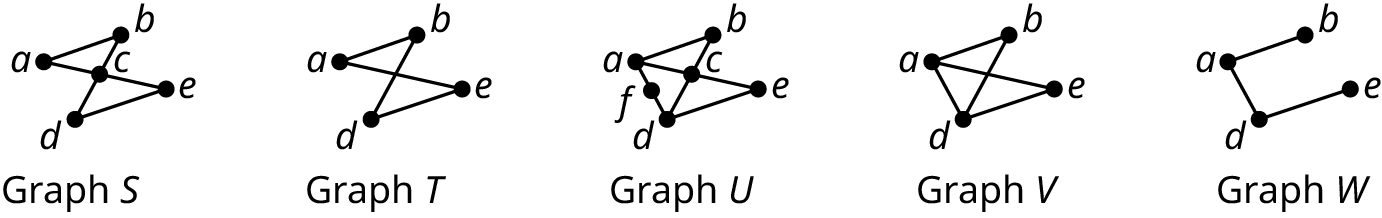1 .
List any graphs that are subgraphs of Graph U.
2 .
List any graphs that are subgraphs of Graph V.
3 .
Explain why Graph T is not a subgraph of Graph S.
4 .
List any graphs that have a quadrilateral cyclic subgraph and name the quadrilaterals.
Use the Sum of Degrees Theorem as needed to answer the following exercises.
5 .
How many edges are in a graph if the sum of the degrees of its vertices is 18.
6 .
Draw two possible graphs to demonstrate that the sum of the degrees of the vertices in a graph with 7 edges is 14. Label the degrees of the vertices.
7 .
What is the sum of the degrees of the vertices of a graph that has 122 edges?
8 .
A graph has 3 vertices of degree 2, 4 vertices of degree 1, and 2 vertices of degree 3. How many edges are in the graph?
9 .
There are 6 vertices and 11 edges in a graph, 2 of degree 5, 1 of degree 4, and 2 of degree 3. What is the degree of the remaining vertex?
10 .
A complete graph has 5 vertices. What is the sum of the degrees of the vertices?
11 .
A complete graph has 120 edges. How many vertices does it have?
Use the figure to answer the following exercises. Identify the graph or graphs with the given characteristic.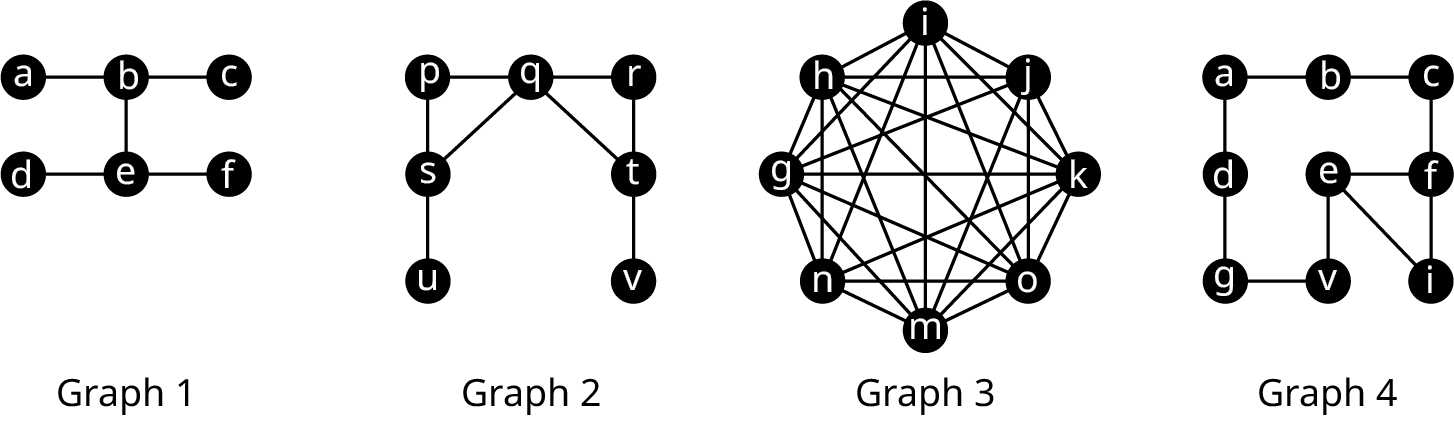12 .
The sum of the degrees of the vertices is 16.
13 .
The graph is complete.
14 .
The graph has no cyclic subgraphs
15 .
The graph contains at least one octagon.
16 .
The graph contains exactly two 3-cycles.
Use the figure to answer the following exercises. Identify the graph or graphs with the given characteristic.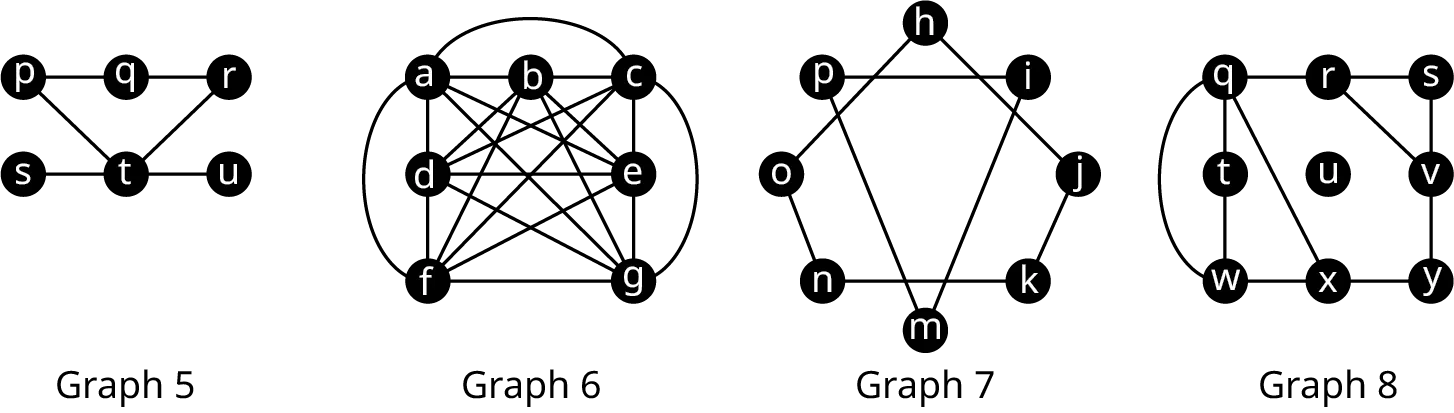17 .
The graph is a subgraph of Graph 6.
18 .
The sum of the degrees of the vertices is 16.
19 .
The graph is complete.
20 .
The graph has no pentagons.
21 .
The graph contains at least one octagon.
22 .
The graph contains exactly two cyclic subgraphs.
23 .
Use Table 12.1 to name a heptagon in Graph 8.
Use the figure to answer the following exercises. Identify the graph or graphs with the given characteristic.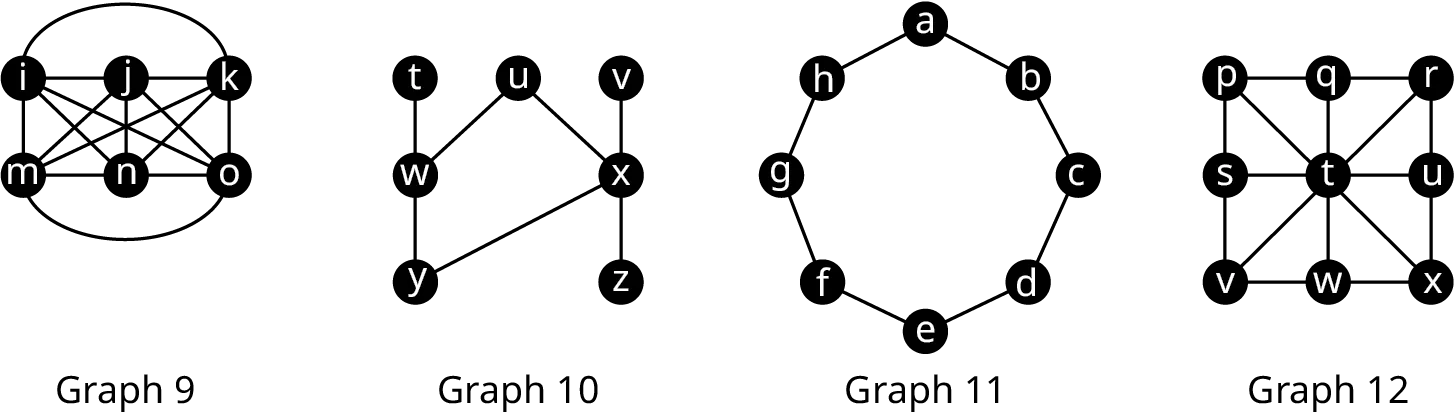24 .
The sum of the degrees of the vertices is 16.
25 .
The graph is complete.
26 .
The graph contains exactly one quadrilateral.
27 .
The graph contains at least one octagon.
28 .
The graph contains exactly one cyclic subgraph.
Use Table 12.1 to answer the following exercises about the below figure.29 .
List every quadrilateral in Graph 12.
30 .
List every quadrilateral in Graph 10.
31 .
Determine the number of quadrilaterals in Graph 9.
Use the figure to answer the following exercises.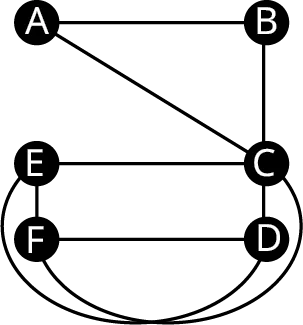32 .
Name five triangles in the graph.
33 .
Identify a clique with more than three vertices in the graph by listing its vertices.
For the following exercises, draw a graph with the given characteristics.
34 .
4 vertices, 6 edges, a subgraph that is a 4-cycle.
35 .
11 vertices, the only cyclic subgraphs are triangles.
36 .
37 .
4 vertices, 4 edges, no cyclic subgraphs
38 .
Complete graph, sum of the degrees of the vertices is 20.
39 .
7 vertices, largest clique has 5 vertices.
40 .
In chess, a knight can move in any direction, but it must move two spaces then turn and move one more space. The eight possible moves a knight can make from a space in the center of a five-by-five grid are shown in the first figure. Draw a graph that represents all the legal moves of a knight on a three-by-three grid starting from the lower left corner as shown in the second figure where the vertices will represent the spaces occupied by the knight and the edges will indicate a move from one space to the next.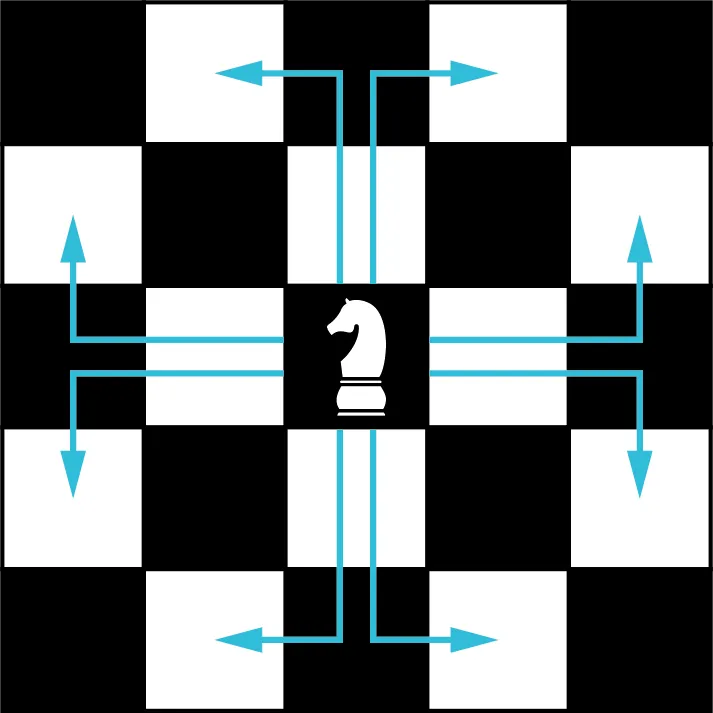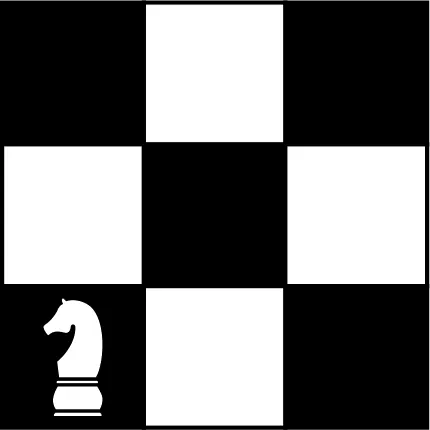41 .
What kind of cycle is the resulting graph you drew for Exercise 40?
42 .
Use Pascal’s triangle to find number of triangles in a complete graph with 14 vertices.
43 .
Do you think that a graph representing network of friends on Facebook is likely to be complete or not? Explain your reasoning.
44 .
Would the graph of a family tree, in which edges represent parent-child lineage, contain any cycles? Why or why not?
45 .
We have seen that the number of triangles in a complete graph with 7 vertices can be calculated using the pattern $(5 + 4 + 3 + 2 + 1) + \left( {4 + 3 + 2 + 1} \right) + (3 + 2 + 1) + \left( {2 + 1} \right) + 1 = 35$. This pattern gets very long for complete graphs with more vertices, but we have seen sums from 1 to a number before, and we had a shortcut! Recall from Example 12.8 that $1 + 2 + 3 + \cdots + (n - 1) = \frac{{n(n - 1)}}{2}$. This makes finding the sum from 1 up to $n - 1$ shorter. We can write each of the sums we need in the form $\frac{{n(n - 1)}}{2}$.
To find the sum from 1 to 5, since $n - 1 = 5$, use $n = 6{:}$ $1 + 2 + 3 + 4 + 5 = \frac{{6(5)}}{2}$
To find the sum from 1 to 4, since $n - 1 = 4$, use $n = 5{:}$ $1 + 2 + 3 + 4 = \frac{{5(4)}}{2}$
To find the sum from 1 to 3, since $n - 1 = 3$, use $n = 4{:}$ $1 + 2 + 3 = \frac{{4(3)}}{2}$
To find the sum from 1 to 2, since $n - 1 = 2$, use $n = 3{:}$ $1 + 2 = \frac{{3(2)}}{2}$
To find the sum from 1 to 1, (Sounds silly, but it helps to see the pattern!) since $n - 1 = 1$, use $n = 2{:}$ $1 = \frac{{2(1)}}{2}$
Use these to rewrite the calculation for the number of triangles in a graph with 7 vertices.
$\begin{gathered} (5 + 4 + 3 + 2 + 1) + \left( {4 + 3 + 2 + 1} \right) + (3 + 2 + 1) + \left( {2 + 1} \right) + 1 \hfill \\ = \underbrace 1_{\frac{{2(1)}}{2}} + \underbrace {(1 + 2)}_{\frac{{3(2)}}{2}} + \underbrace {(1 + 2 + 3)}_{\frac{{4(3)}}{2}} + \underbrace {(1 + 2 + 3 + 4)}_{\frac{{5(4)}}{2}} + \underbrace {(1 + 2 + 3 + 4 + 5)}_{\frac{{6(5)}}{2}} \hfill \\ = \frac{{2(1)}}{2} + \frac{{3(2)}}{2} + \frac{{4(3)}}{2} + \frac{{5(4)}}{2} + \frac{{6(5)}}{2}\quad\quad\quad\quad\quad\quad\quad\quad\quad\quad\quad\quad\quad \hfill \\ {\text{ = }}\underbrace {\frac{{1\left( 2 \right) + 2\left( 3 \right) + 3\left( 4 \right) + 4\left( 5 \right) + 5\left( 6 \right)}}{2}}_{{\text{The number of triangles in complete graph with 7 vertices}}{\text{.}}} = 35\quad\quad\quad\quad\quad\quad\,\, \hfill \\ \end{gathered}$
Similarly, for the number of triangles in a complete graph with 8 vertices, instead of $(6 + 5 + 4 + 3 + 2 + 1) + (5 + 4 + 3 + 2 + 1) + \left( {4 + 3 + 2 + 1} \right) + (3 + 2 + 1) + \left( {2 + 1} \right) + 1 = 56$
use the shorter formula $\frac{{1\left( 2 \right) \,+\, 2\left( 3 \right) \,+\, 3\left( 4 \right) \,+\, 4\left( 5 \right) \,+\, 5\left( 6 \right) \,+\, 6(7)}}{2} = 56$.
Use this pattern to find the number of triangles in a complete graph with 10 vertices. In which row and entry of Pascal’s triangle can you also find this result?
46 .
Use the fact that the sum from 1 to $n - 1$ is $\frac{{n(n - 1)}}{2}$ to write a formula for the number of triangles in a complete graph with n vertices.
Order a print copy

As an Amazon Associate we earn from qualifying purchases.